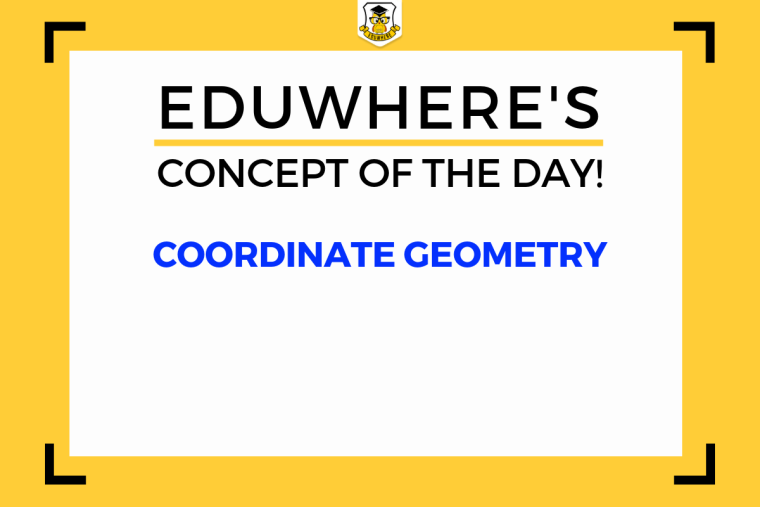## Eduwhere's Concept: Coordinate GeometryCoordinate Geometry questions asked in the Quantitative Aptitude section in the CAT examination can be extremely easy if you have your concepts clear. Let’s take a look at some of the vital concepts related to Coordinate Geometry which might help you in your upcoming examination.

Visit Eduwhere to take online mock tests for your upcoming exams.

All the points are located in the coordinate plane when we talk about coordinate geometry. It is all about the x-axis and y-axis.

Some basic concepts of Coordinate Geometry:

Origin is the point of intersection of the x and y-axis where both x and y are 0.

Always remember that on the right-hand side of the x-axis, the values are positive and on the left-hand side of the x-axis, the values are negative.

The same applies to y-axis where all the values located above the origin are positive and those located below the origin are negative.

A set of two numbers is used to locate a point on the plane which is written as the location on the x-axis initially, followed by its location on the y-axis. In union, the two will determine a unique position on the plane.

Here is a bit more you should know to solve Coordinate Geometry problems.

Focus on all the four mentioned formulas:

Section Formula for Internal division

Section Formula for External division

Midpoint Formula

Distance Formula

1

1383